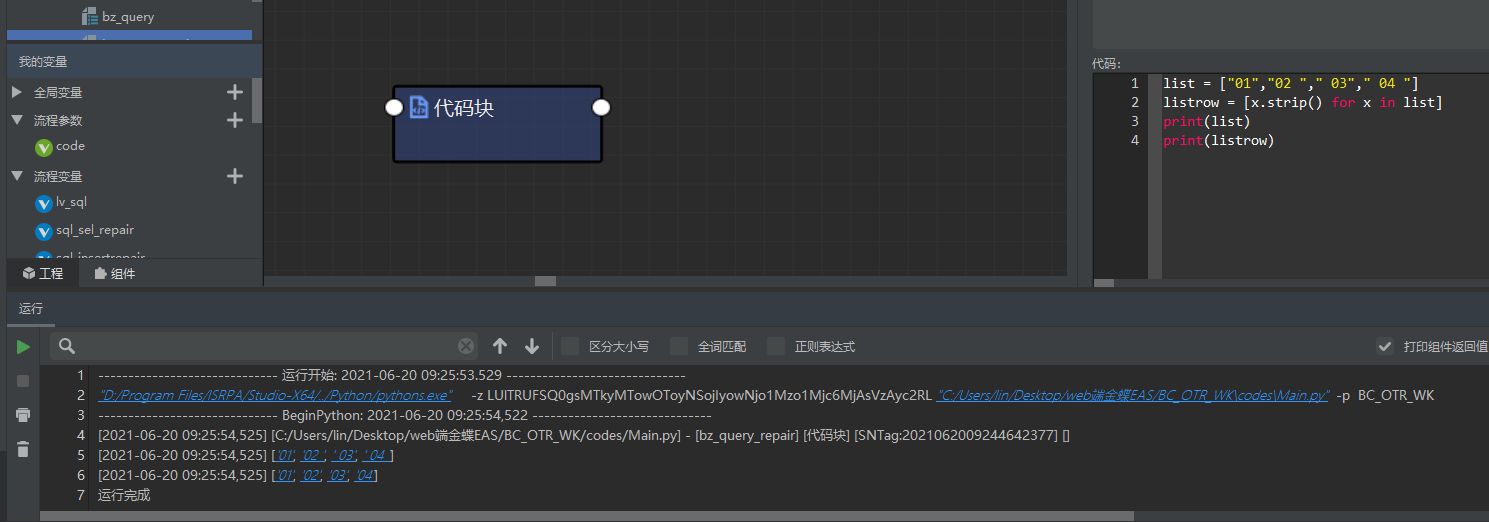# 对 python 数据的处理方法

#### 一. 对列表进行处理

1. 对列表中的所有元素进行去空格`````` listrow = [x.strip() for x in ["01","02 "," 03"," 04 "]]
``````
2. 将列表元素转换为元组

``````tupl=tuple(list1)
``````

#### 二. 对 dataframe 进行处理

1. dataframe 遍历行数据
`````` for index, row in df.iterrows():
#index为下标
print(index)
#row为数据行
print(row)
``````
2. dataframe 取某一行
``````#取第一行数据，下标从0开始
df.iloc
``````
3. dataFrame 空值处理

``````#指定填充的值
print(df.fillna(0))
``````

#### 三. 字符串拼接的三种方式

1. 字符串拼接
``````lvurl = "#s-top-left > A:nth-of-type("+str(lvindex)+")"

``````
2. 格式化字符串
``````lvurl = "#s-top-left > A:nth-of-type({})".format(str(lvindex))
``````
3. 占位符

``````lvurl = "#s-top-left > A:nth-of-type(%s)"%str(lv_index)
``````